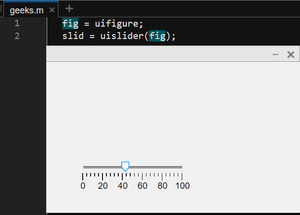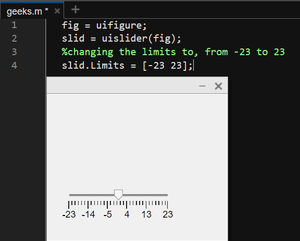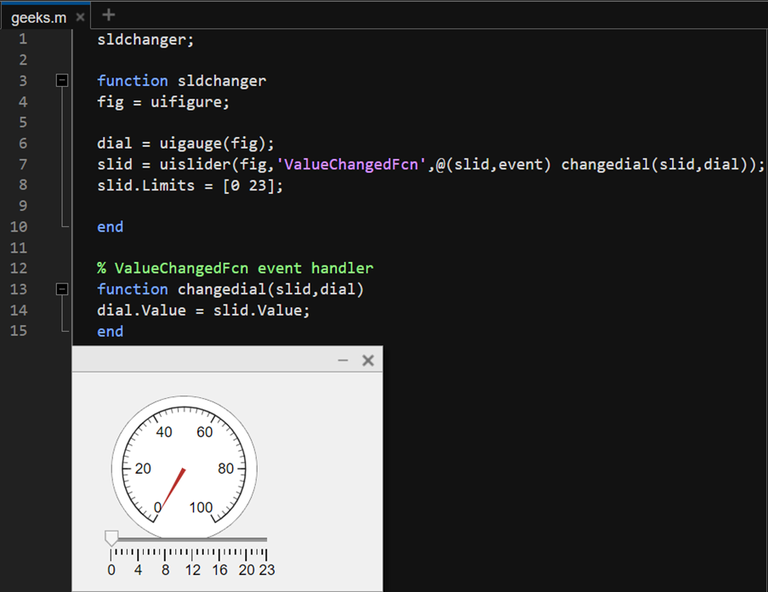GFG App
Open AppBrowser
Continue

# Create a Slider Component in MATLAB

A slider component is a graphical interface that allows the end users to select discrete values in the range of the slider component. MATLAB provides built-in functionalities to create slider components in a MATLAB figure component.

This article will explain how to create a slider component in MATLAB and the various options available with it.

## Creating Slider Component

The uislider function creates a new slider object in a new uifigure component unless no parent figure component exists. The syntax for the same is:

slider_name = uislider(<optional parameters>)

The syntax for uislider is simple. If no parent figure exists then, MATLAB creates a new figure component and then adds a slider to it. If a parent figure is given to the function then, it will create the slider in that particular figure.

Example 1:

## Matlab

 `% creating the parent figure ` `fig = uifigure; ` ` `  `% adding the slider component ` `slid = uislider(fig);`

Output:As can be seen, the slider component has been added to the figure.

## Changing the Limits of the Slider

As seen in the previous example, the slider took default values from 0 to 100. However, we can change the same to any desired limits using the following method.

Example 2:

## Matlab

 `% Code ` `fig = uifigure; ` `slid = uislider(fig); ` `%changing the limits to, from -23 to 23 ` `slid.Limits = [-23 23];`

By using the slider_object.Limits property of uislider, we can change the limits of the slider to any desired value; range given as a vector.

Output:## Using the Slider Value

So far, the slider does not manipulate any actual data, it is just a graphical slider. To use the slider values, we need to add the ‘ValueChangedFcn’ property of the slider. This property uses the event handler of MATLAB apps, event. We use the event, and use the values from the slider. See the following example to understand the same.

Example 3:

## Matlab

 `sldchanger; ` ` `  `% function to generate slider and a  ` `% dummy dial to show changed values ` `function` `sldchanger ` `fig = uifigure; ` ` `  `dial = uigauge(fig); ` ` `  `% Creating the slider and assigning  ` `% its values to the dial/gauge created above ` `slid = uislider(fig,``'ValueChangedFcn'``,@(slid,event) changedial(slid,dial)); ` ` `  `% setting limits to 0 to 23 ` `slid.Limits = [0 23]; ` `end` ` `  `% ValueChangedFcn event handler ` `function` `changedial(slid,dial) ` ` `  `% changing dialer values to slider  ` `% values after each slide ` `dial.Value = slid.Value; ` `end`

Output:In the above code, we create a slider component with limits from 0 to 23 and assign its changed values to a uigauge dial component. The same is done by creating a function handle to assign the dial its values the same as the slider pointing value, and then passing that function handle to the ‘ValueChangedFcn’ parameter.

My Personal Notes arrow_drop_up